• 交叉熵代价函数
• 四种称为规范化的⽅法（L1 和 L2 规范化，弃权和训练数据的⼈为扩展）
• 更好的权重初始化⽅法
• 如何选择神经网络的超参数

## 交叉熵代价函数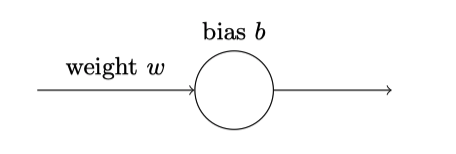$$\frac{1}{1+\exp(-\sum_j w_j x_j-b)}$$

0.6 和 0.9带入，即可得到输出为：0.82，很显然和目标0相差甚远，那么就只能继续寻找最优解了，随着迭代期的增加，神经元的输出、权重、偏置和代价的变化如下⾯⼀系列图形所⽰：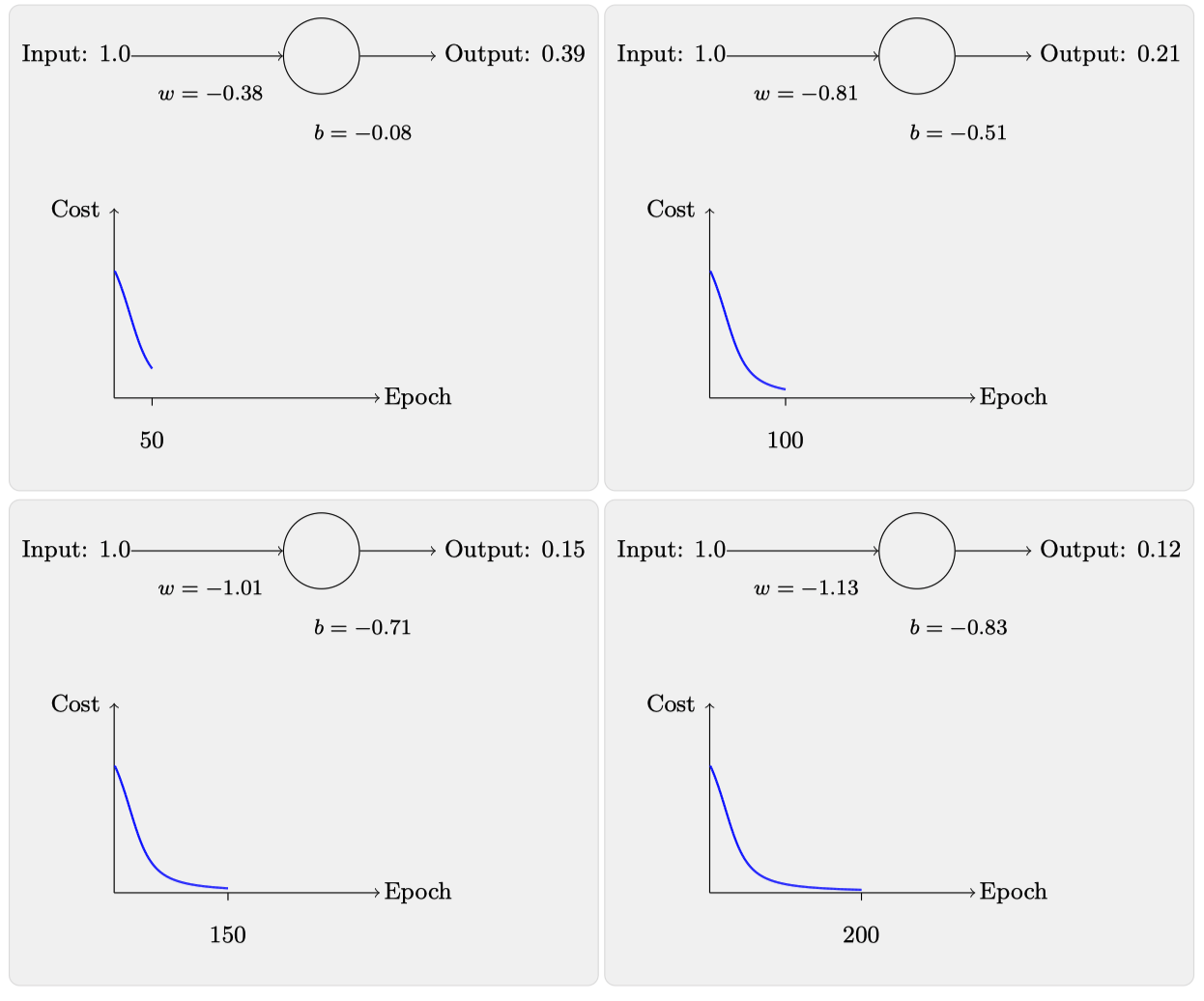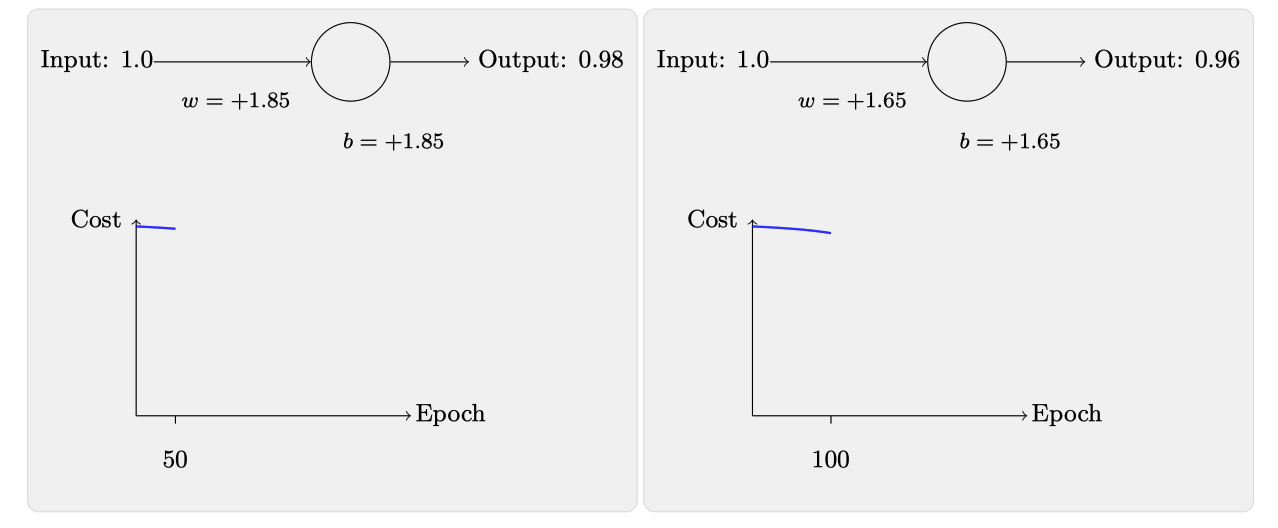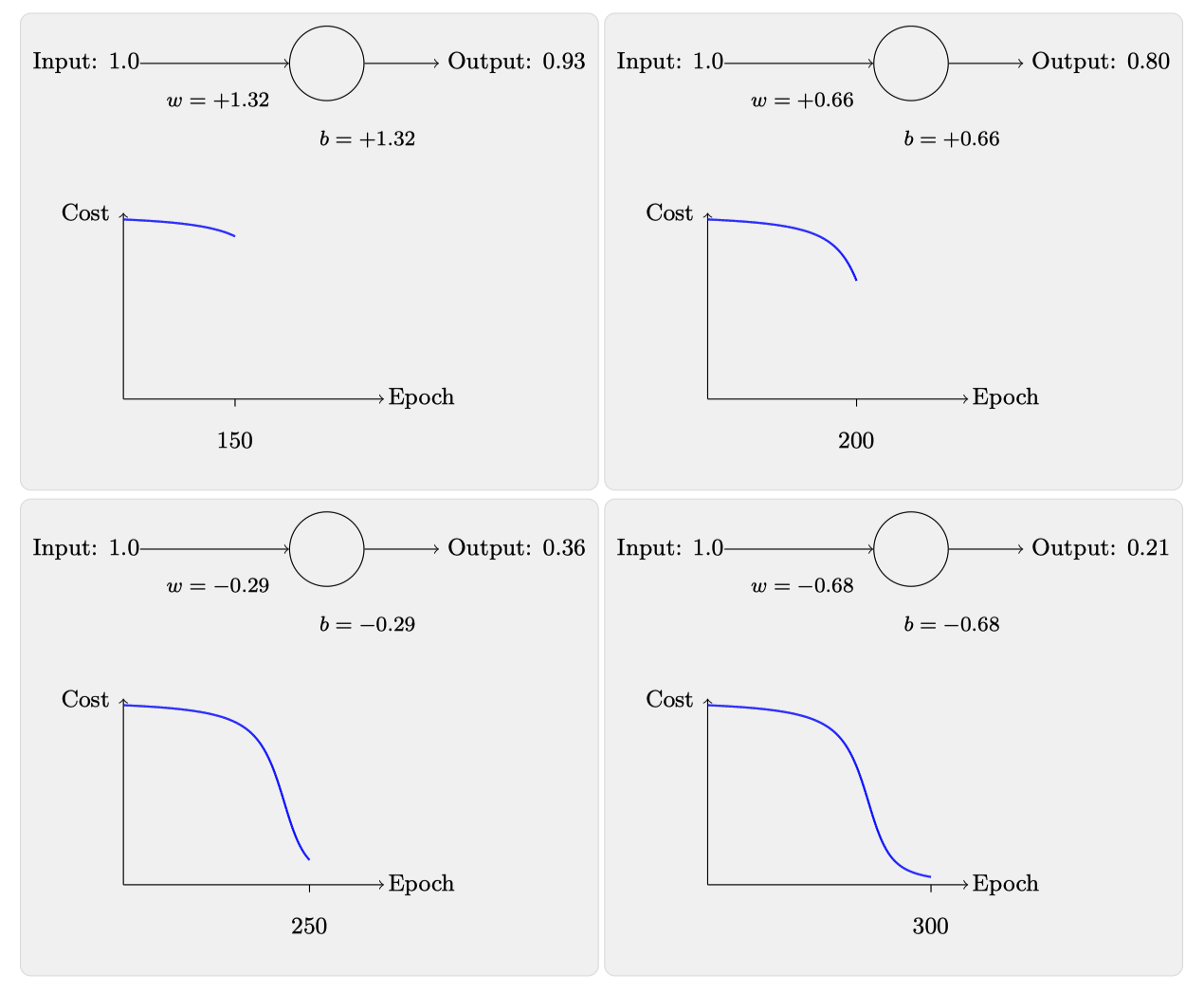• $x=1$
• $y=0$

$$C(w,b) \equiv \frac{1}{2n} \sum_x | y(x) - a|^2$$

• w 表⽰所有的⽹络中权重的集合
• b 是所有的偏置
• n 是训练输⼊数据的个数
• a 是表⽰当输⼊为x时输出的向量，可以理解为output

$$C=\frac{(y-a)^{2}}{2}$$

• $x=1$
• $y=0$
• $a=\sigma(z)$
• $z=wx+b$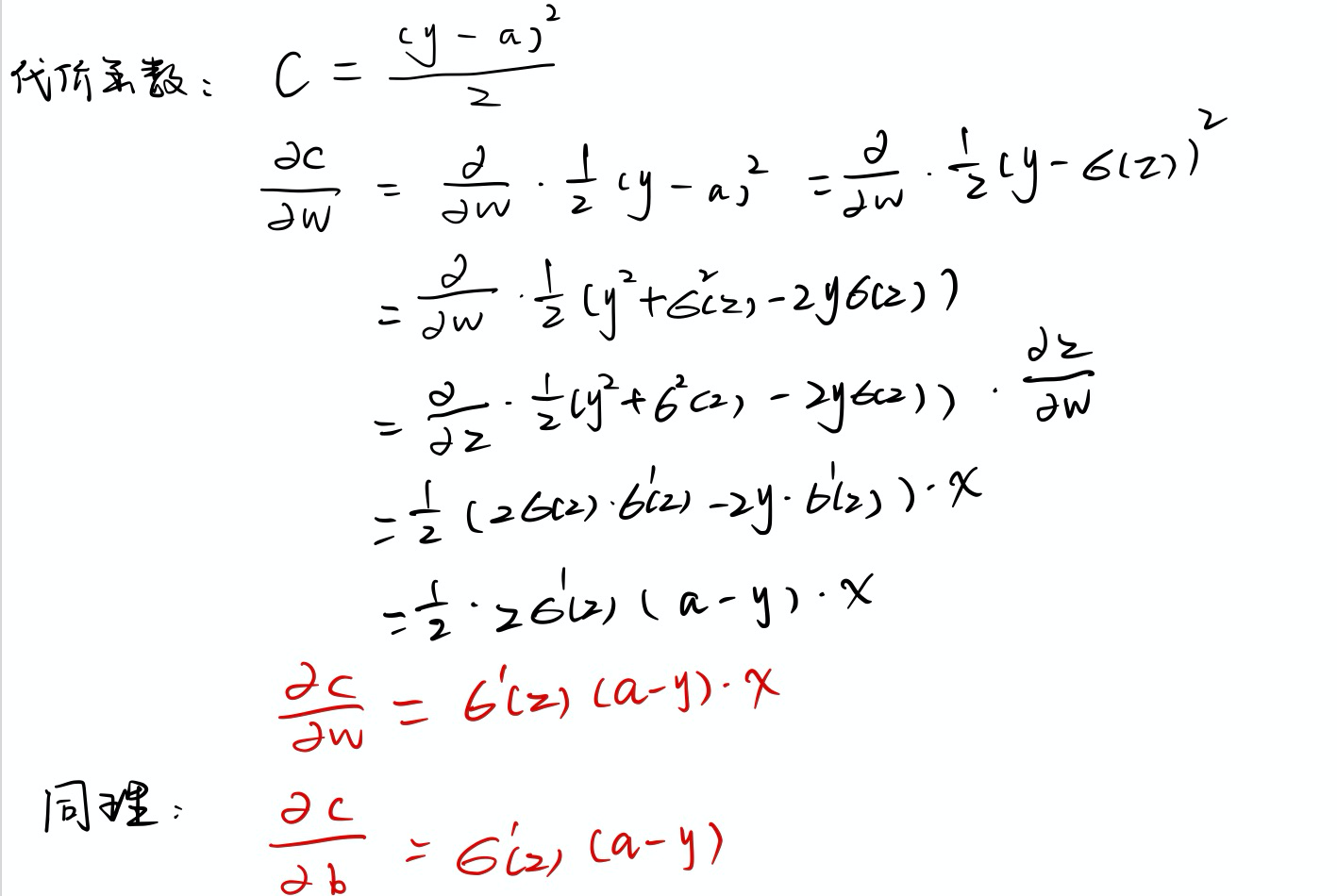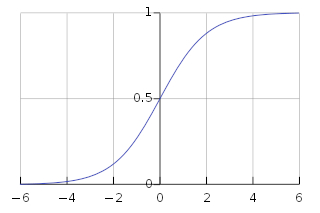### 引⼊交叉熵代价函数• $a=\sigma(z)$
• $z=\sum_{j} w_{j} x_{j}+b$

$$C=-\frac{1}{n} \sum_{x}[y \ln a+(1-y) \ln (1-a)]$$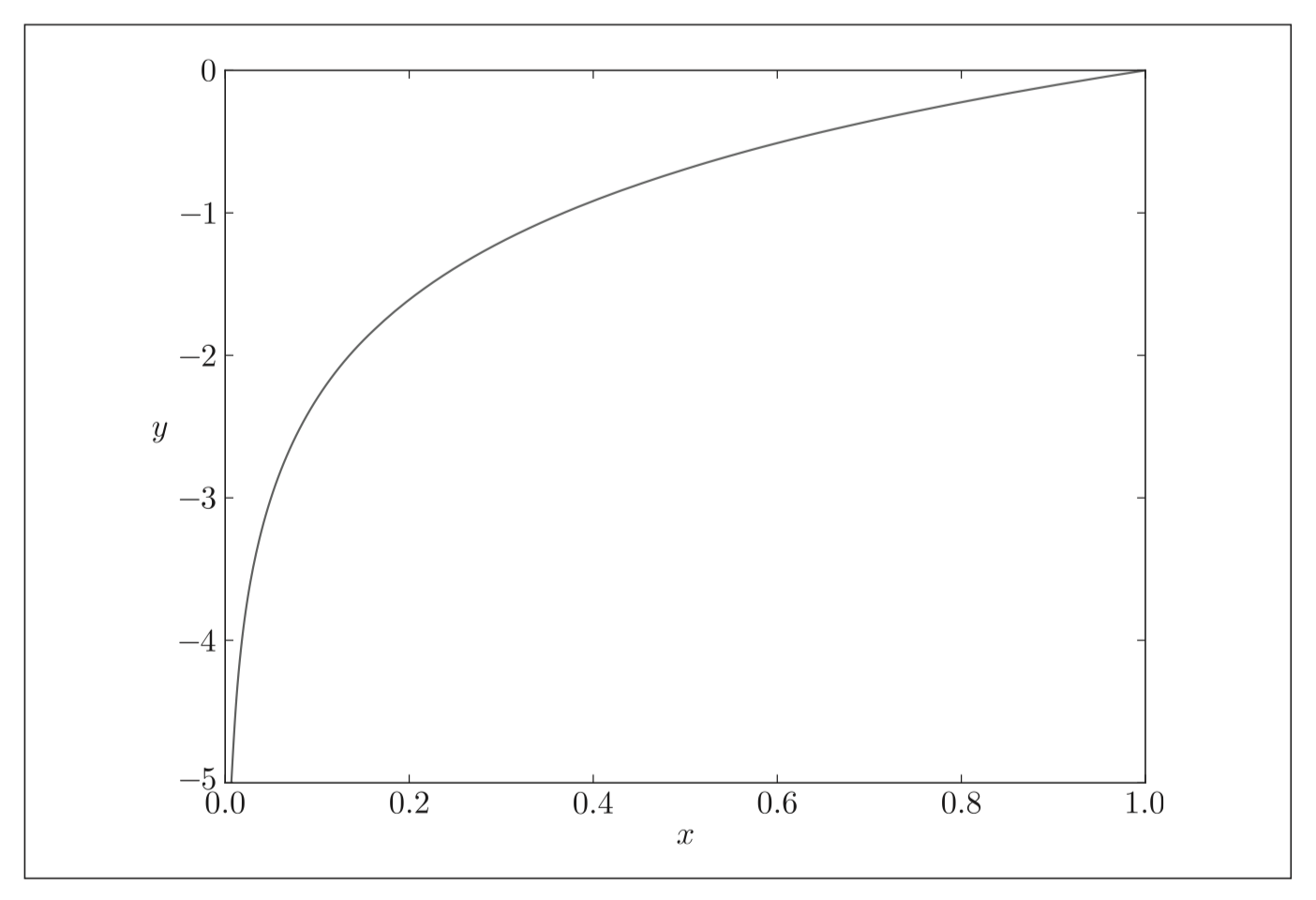• 总是非负的
• 实际输出值和目标输出值之间的差距越小，最终的交叉熵的值接越低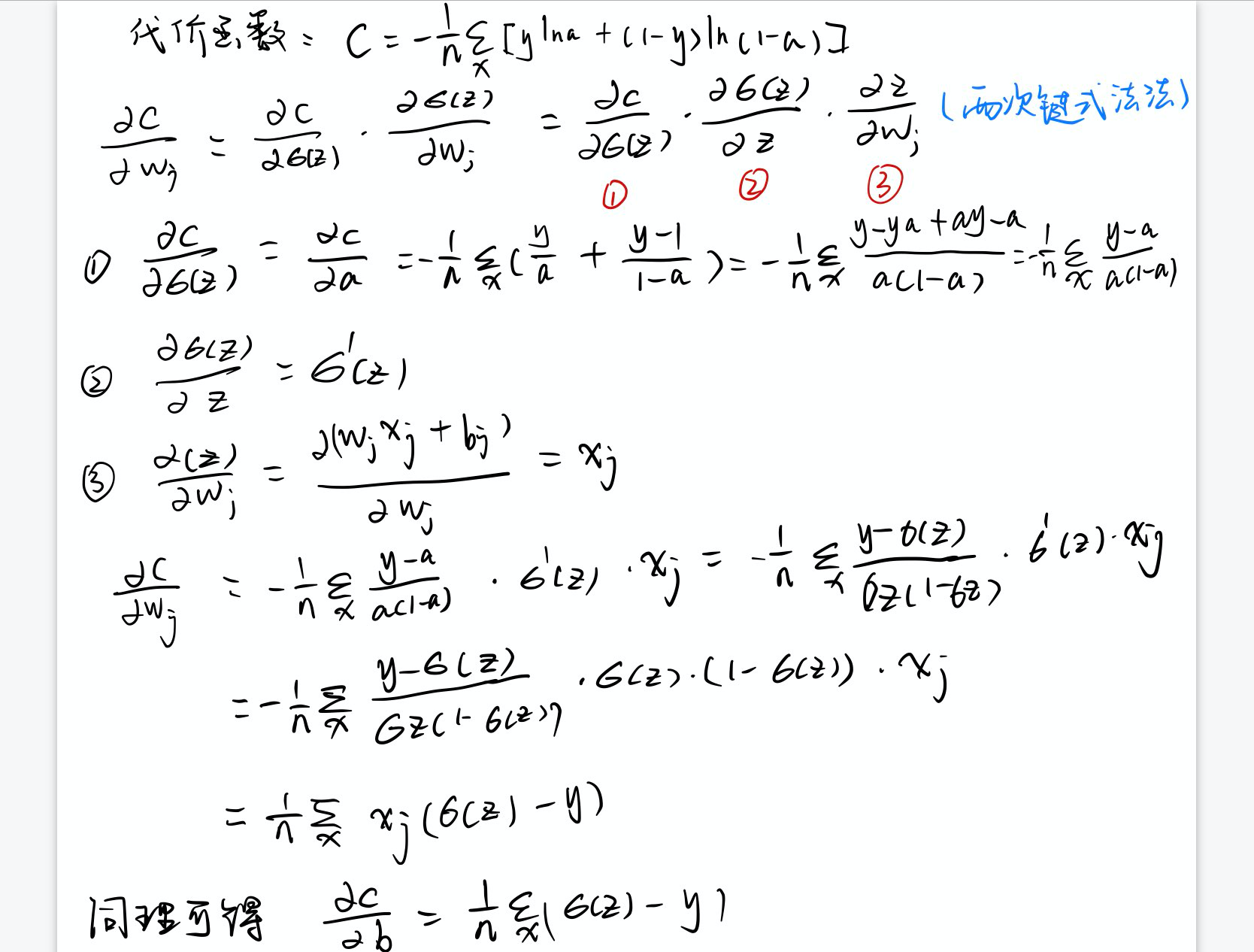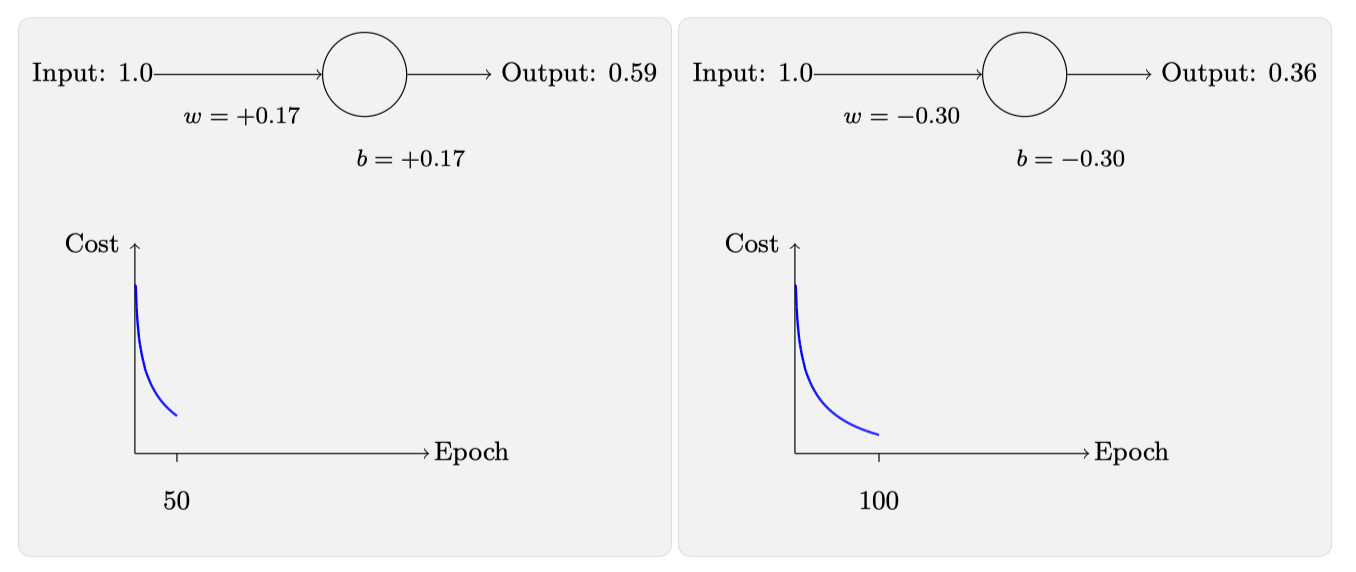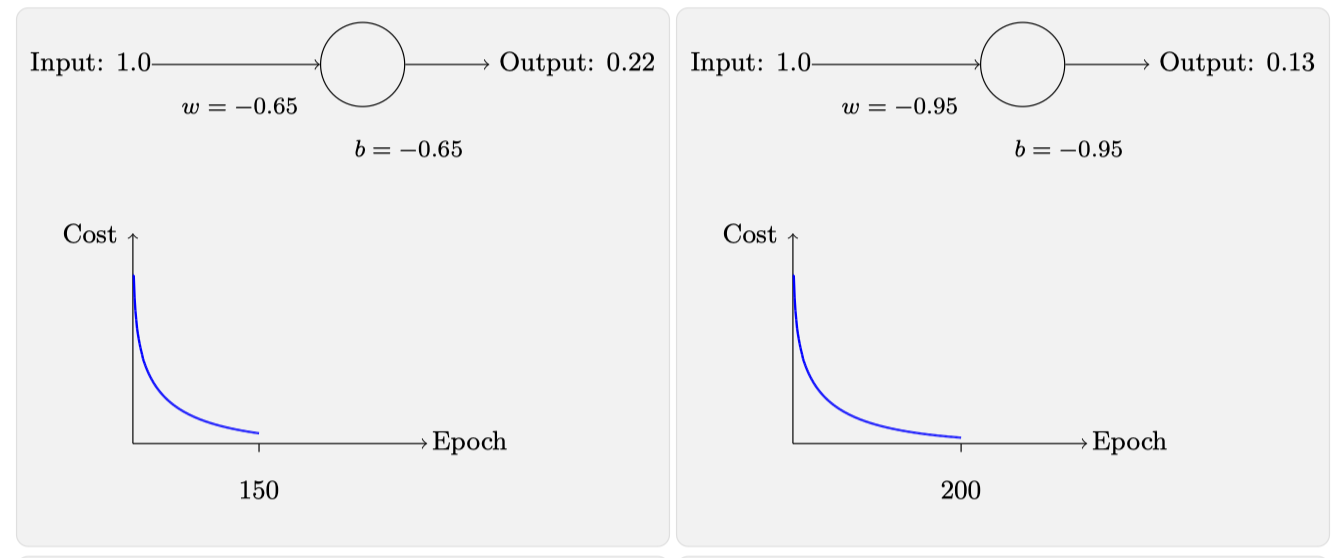• 误差越大，学习速度越快
• 迭代周期明显变小

$$C=-\frac{1}{n} \sum_{x} \sum_{j}\left[y_{j} \ln a_{j}^{L}+\left(1-y_{j}\right) \ln \left(1-a_{j}^{L}\right)\right]$$

### 交叉熵的含义？源⾃哪⾥？

\begin{aligned} \frac{\partial C}{\partial w} &=(a-y) \sigma^{\prime}(z) x=a \sigma^{\prime}(z) \ \frac{\partial C}{\partial b} &=(a-y) \sigma^{\prime}(z)=a \sigma^{\prime}(z) \end{aligned}

\begin{aligned} \frac{\partial C}{\partial w_{j}} &=x_{j}(a-y) \ \frac{\partial C}{\partial b} &=(a-y) \end{aligned}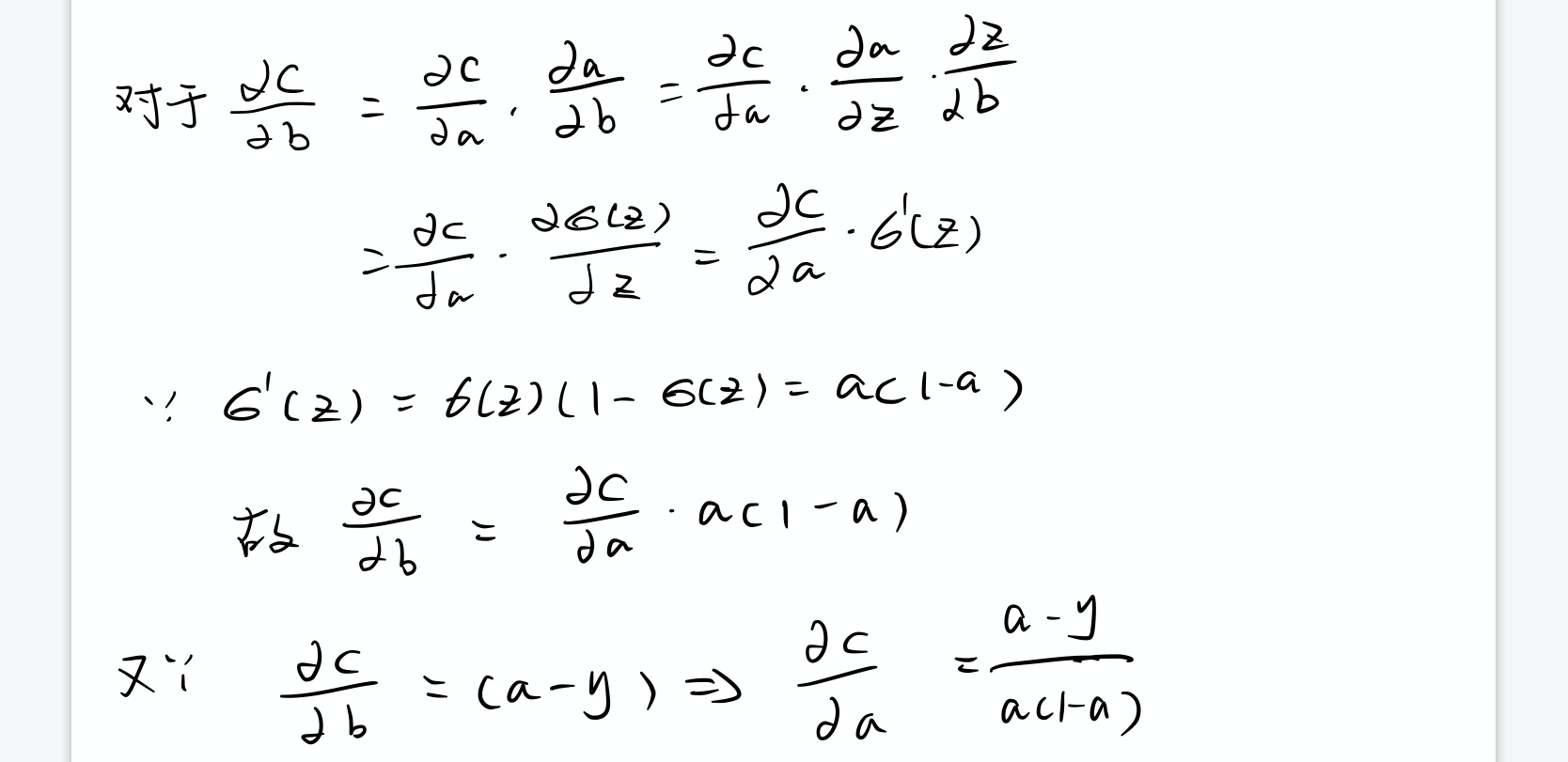$$C=-[y \ln a+(1-y) \ln (1-a)]+\mathrm{constant}$$

$$C=-\frac{1}{n} \sum_{x}[y \ln a+(1-y) \ln (1-a)]+\mathrm{constant}$$

## 过度拟合和规范化

### 规范化

$$C=-\frac{1}{n} \sum_{x j}\left[y_{j} \ln a_{j}^{L}+\left(1-y_{j}\right) \ln \left(1-a_{j}^{L}\right)\right]+\frac{\lambda}{2 n} \sum_{w} w^{2}$$

### 为何规范化可以帮助减轻过度拟合

⼩的权重在某种程度上，意味着更低的复杂性，也就对数据给出了⼀种更简单却更强⼤解释，因此应该优先选择。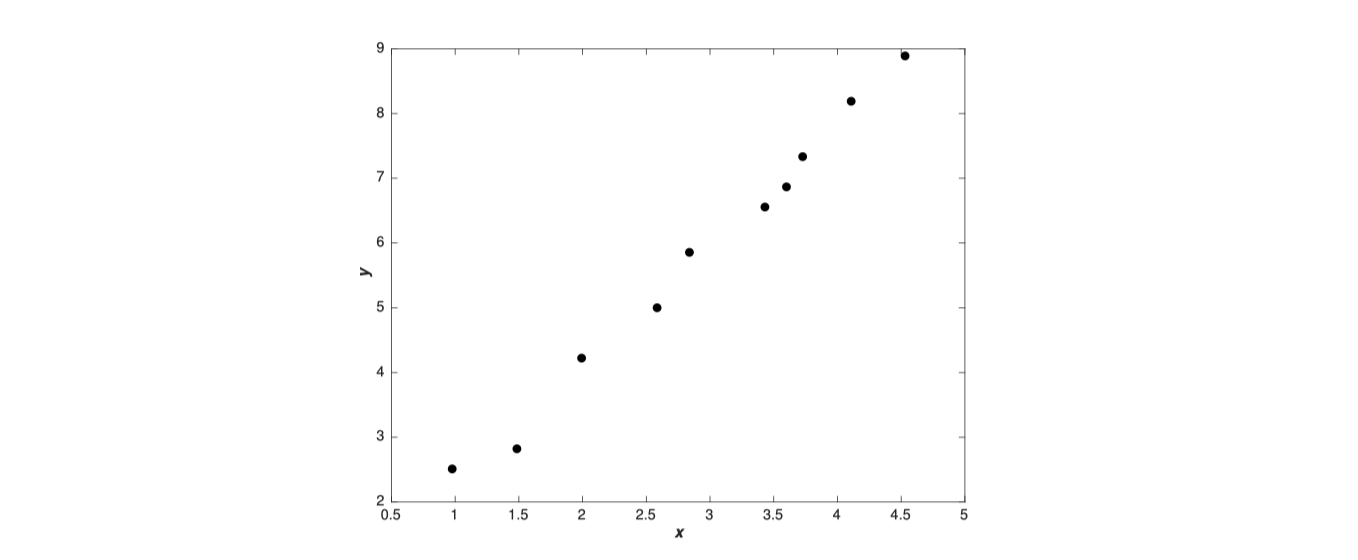$$y=a_{0} x^{9}+a_{1} x^{8}+\ldots+a_{9}$$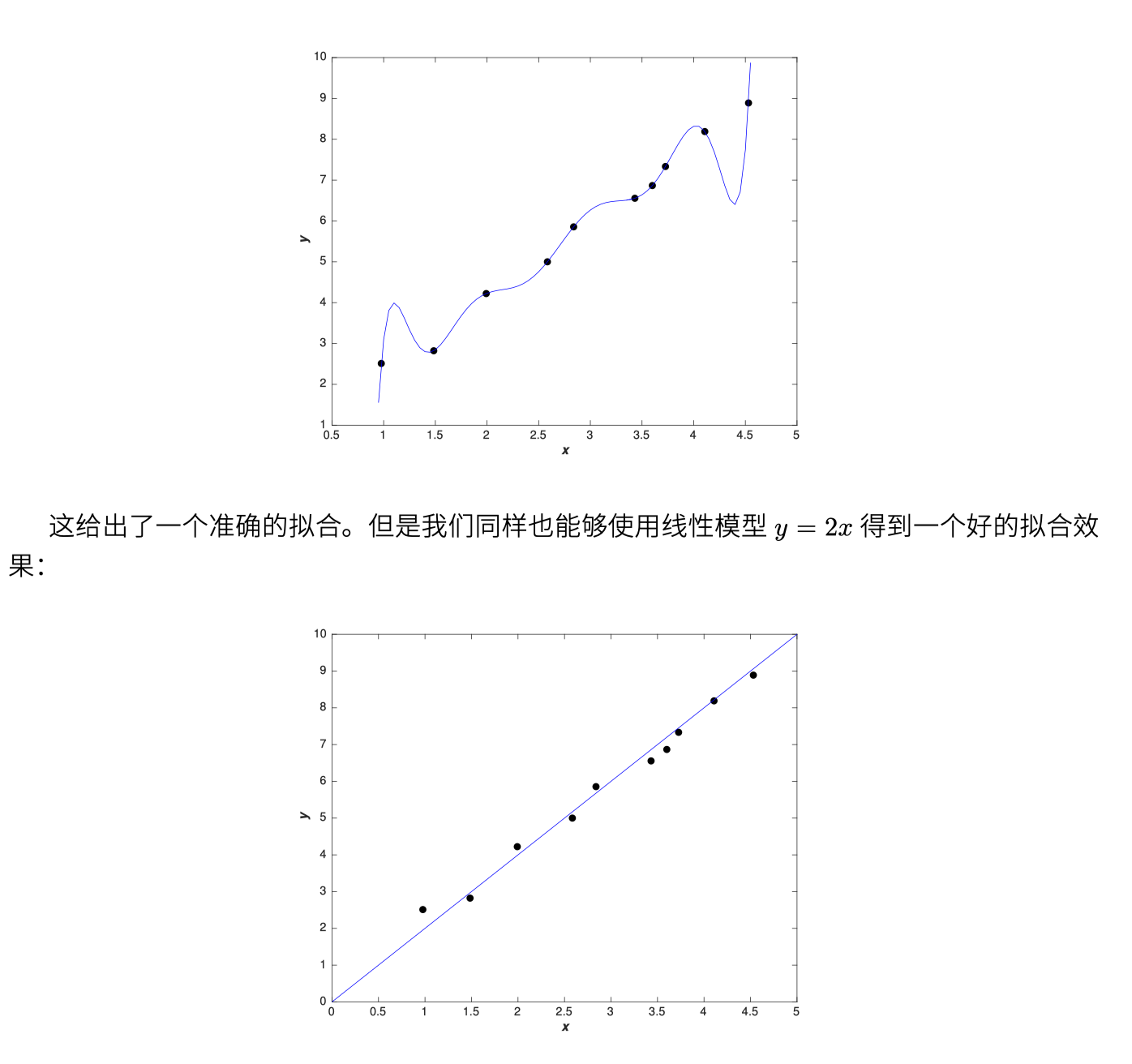### 规范化的其他技术

• L1规范化
• 弃权（Dropout）
• 人为增加训练样本

L1规范化：这个⽅法是在未规范化的代价函数上加上⼀个权重绝对值的和：

$$C=C_{0}+\frac{\lambda}{n} \sum_{w}|w|$$

L1规范化倾向于聚集⽹络的权重在 相对少量的⾼重要度连接上，⽽其他权重就会被驱使向0接近

⼈为扩展训练数据：数据量大到一定程度可以弥补算法的差距，没啥好说的。

## 如何选择神经⽹络的超参数

• 宽泛策略：
• 将复杂的问题简单化，比如先减少目标类别，简化网络结构
• 在问题简化后可以方便地进行对超参数进行调节，快速调试是哪个超参数有问题
• 学习速率：可以观察在某些学习速率下，迭代周期和训练代价的折线图变化情况，主要观察训练代价开始下降的学习率，学习速率可以变成可变的，在不同的迭代次数用不同的学习率
• 早停法：这是我个人用得比较多的方法，判断验证集的准确率以及训练代价，比如效果不再提升，就可以考虑停止迭代，输出对应模型
• 正则化参数：开始时不包含正则化（λ = 0.0），确定 η 的值，使⽤确定出来的 η，我们可以使⽤验证数据来选择好的 λ。从尝试 λ = 1.0 开始，然后根据验证集上的性能按照因⼦ 10 增加或减少其值。⼀旦我已经找到⼀个好的量级，你可以改进 λ 的值。这⾥搞定后，你就可以返回再重新优化 η。
• 自动技术：网格搜索